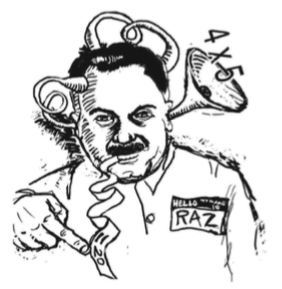# Raz’s Magic Multiplying Machine

Here is a question you don’t hear very often: What does it feel like to experience multiplication in our bodies? It’s a strange question because our typical exposure to multiplication is numerical. I give you two numbers—say, 3 and 5—and you tell me their product, 15. But multiplication need need not be so static and concrete.

Back in early September of 2001, Steve Rasmussen and I were waiting for a delayed plane back from Penn State. As we sat at the airport, we discussed what it would mean to design a Sketchpad model in which students could experience the behavior of multiplication rather than the specifics of multiplying two particular numbers.

Our brainstorming led to the Web Sketchpad model below (and here), which I titled Raz’s Magic Multiplying Machine. Two points, labeled a and b, sit on a number line along with their product, a·b. You can drag either point a or b continuously along the number line and view the effect on the product. In effect, both a and b are independent variables while a·b is the dependent variable.

When we give this multiplication model to students we leave the questions open ended. We ask students to drag points a and b and take note of any interesting behaviors of a, b, and their product, a·b. Here are some sample observations:

• I thought that the product of a and b must be larger than both a and b. But I can see that’s not always true. When a is between 0 and 1 and b is greater than 0,  then b is greater than a·b. And when both a and b are between 0 and 1, then a·b is less than both of them.
• I’m looking for places where a and a·b have the same value. When b = 1, a and a·b are equal. In fact, when I drag a, the product a·b stays right underneath it. It’s as if they’re locked together. I also see that if I set a to 0 and drag b, then a·b stays at 0.
• If I leave a in place and drag b, I can compare how fast b and a·b are moving. When a = 2, for example, I see that a·b  is moving twice as fast as b. And when a = –1, then b and a·b are moving in opposite directions at the same speed. It’s like they’re reflections of each other across 0.

It’s also illuminating to use this number line model to explore why a negative number multiplied by a positive number is negative.  Start with a and b considerably to the right of 0. Now, slowly drag a to the left. As you do, a·b moves smoothly to the left as well, getting smaller and smaller; The product equals 0 as a passes through 0 and then continues on its way to the left into negative territory when a becomes negative. A similar continuity argument helps to explain why the product of two negative values is positive. Start with a to the right of 0 and b to the left of 0 and slowly drag a to the left, passing through 0 on its way.

Finally, if you go to the second page of the websketch (press the arrow in its lower-right corner), you’ll see a number line without any markings. Here the challenge is to drag a and b and use the behavior of a·b to pinpoint the locations of 0 and 1. Students drag the orange arrows in the top-right corner of the websketch to indicate the locations of 0 and 1 and then press Show 0 and 1 to check their predictions. Note that this is a way more stimulating approach to introducing the properties of 0 and 1 as opposed to stating blandly that for all a, a·1 = a and a·0 = 0.

Incidentally, when Raz’s Magic Multiplying Machine appeared in Exploring Algebra 1 with The Geometer’s Sketchpad, Key’s art department prepared the illustration below to accompany it. It’s a tribute to that mad math inventor, Steve Rasmussen!

P.S. When this site began in 2010, it was the official blog of Key Curriculum Press. We’ve kept the domain blog.keycurriculum.com as the home of this blog, but since Key no longer exists,  it is time to change it. Since our blog is Sine of the Times, it is only fitting that our new home be sineofthetimes.org. Same content, new address!

An annotated list of all our elementary-themed blog posts is here.